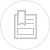# customary, definitions and formulae

## alternating current

#### power factor or cos Ø

Intensity and an alternating current voltage will rarely be in phase. The cosinus of the angle formed by the two intensity voltage vectors is called the power factor.

When a self-induction is incorporated into a circuit, it steps the intensity back from the voltage; when a capacitance is incorporated, it has the opposite effect.

The active power is decreased in both cases (figure 45).

In the case of an industrial sinusoidal current (general case) :

• Ia = I cos Ø is the active current;
• Ir = I sin Ø is the reactive current.

#### apparent, active, reactive power

I is the intensity read on an ampere metre, U the main voltage between phases, for a three phase supply.

u is the mains voltage between phase and neutral, for a single phase supply, power is detailed in table 75.

The apparent power is expressed in volt-amperes (VA).

The active power is expressed in Watts (W).

The reactive power is expressed in reactive volt-amperes (VAr).

A poor cos Ø is detrimental to both the energy user and to the energy supplier. It requires large conduit sections and higher apparent power levels for alternators and transformers.

#### circuit impedance

Circuit impedance is the resultant of the ohmic resistance and the circuit’s reactance.

R = resistance,

X = reactance (inductive, capacitive).

Amount of heat given off by a circuit having a resistance R and crossed by a current I for one second.